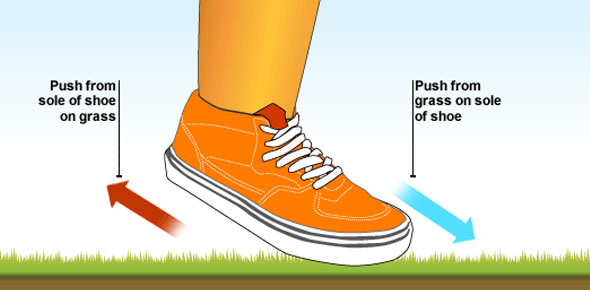# Laws Of Motion Quiz

18 Questions | Attempts: 368
ShareSettingsQuiz yourself on how well you understand Newton's Laws of Motion

• 1.
How long will an object remain at rest, according to Newton's First Law of Motion?
• A.

It can't rest.

• B.

Until noon.

• C.

Until an unbalanced force occurs.

• D.

As long as there are unbalanced forces acting on it.

• 2.
What is inertia?
• A.

The tendency of an object to continue doing what it is currently doing.

• B.

The opposing force of gravity.

• C.

Another name for unbalanced forces.

• D.

The mass of an object.

• 3.
Which of Newton's Laws describes the amount of force applied to accelerate an object's mass?
• A.

Newton's First Law

• B.

Newton's Second Law

• C.

Newton's Third Law

• D.

Newton's Fourth Law

• 4.
For every ________ there is an equal and opposite _____________.
• A.

Reaction, action

• B.

Action, action

• C.

Reaction, reaction

• D.

Action, reaction

• 5.
The amount of force needed to move an object is measured in...
• A.

Newtons

• B.

Kilometers

• C.

Seconds

• D.

Grams

• 6.
Gravity and magnetism are examples of what kind of forces?
• A.

Frictional

• B.

Non-contact

• C.

Contact

• D.

Momentum

• 7.
A person is measured on the moon, and on Earth.  Which statement would be true?
• A.

The person's mass on Earth would be double on the moon.

• B.

The person's mass on the the moon, would be twice that on Earth.

• C.

The person would have mass on Earth but not on the moon.

• D.

The mass would be the same on the moon as it is on Earth.

• 8.
A person is measured on the moon and on Earth.  Which statement would be true?
• A.

The mass would be the same on the moon as it is on Earth, but the weight would be more on the moon than it is on Earth.

• B.

The weight would be the same on the moon as it is on Earth, and the mass would be more on the moon than it is on Earth.

• C.

The mass and weight would be the same on the moon as it is on Earth, because mass is another name for weight.

• D.

The mass would be the same on the moon as it is on Earth, but the weight would be more on Earth than it is on the moon.

• 9.
The amount of force that gravity has on an object is affected by what factors?
• A.

The object's mass.

• B.

The object's distance from the gravitational force.

• C.

The object's height.

• D.

Both A and B.

• 10.
Why is it harder to push a van filled with bowling balls than it is to push van filled with basketballs?
• A.

The mass of the car is greater than the mass of the basketball.

• B.

The mass of the bowling balls is greater than the mass of the basketballs.

• C.

The van with the basketballs is bigger than the van with the bowling balls.

• D.

It should be equally as hard to push the two vans.

• 11.
How could you tell if a moving object is receiving an unbalanced force?
• A.

It might speed up.

• B.

It might slow down.

• C.

It might change direction.

• D.

It might do any of the three things mentioned above.

• 12.
A plane flew from NY to California in 4 hours.  It took 4 1/2 hours to return to NY.  Which of the following is true:
• A.

The plane flew faster to NY than it flew to California.

• B.

The plane flew faster to California than it flew to NY.

• C.

The plane flew the same speed in both directions.

• D.

The plane had fewer passengers going to NY than it did to California.

• 13.
Why is it difficult to scoot a bed from one end of the room to the other?
• A.

Momentum

• B.

Gravity

• C.

Friction

• D.

Deceleration

• 14.
Which of Newton's Laws is demonstrated by a ball rolling to a wall then stopping?
• A.

Newton's First Law

• B.

Newton's Second Law

• C.

Newton's Third Law

• D.

Newton's Law of Acceleration

• 15.
Why do you lean towards the left when a car turns right?
• A.

Because of inertia

• B.

Because of gravity

• C.

Because of buoyancy

• D.

Because of velocity

• 16.
How can you tell if an object is in motion?
• A.

The object will look different.

• B.

I'll be able to see the forces.

• C.

It will remain in the same position.

• D.

Its position will change in relation to other objects.

• 17.
What is "position"?
• A.

A type of contact force.

• B.

A specific location

• C.

A type of motion

• D.

Another name for friction

• 18.
Blue bumper car, traveling east with a force of 100 N, runs into a yellow bumper car traveling west with a force of 300 N.  Because the net force will be -200 N. one of the cars will be pushed backwards.  Which one?

## Related TopicsBack to top
×

Wait!
Here's an interesting quiz for you.